# 1 About the package

This vignette describes comprehensively how the R package rehh can be applied to perform whole genome scans for footprints of selection using statistics related to Extended Haplotype Homozygosity (EHH) (Sabeti et al. 2002). The vignette Examples in detail explains basic usage and methodology with the help of two tiny artificial data sets.

The package accepts multi-allelic genetic markers as input. Typically, albeit not necessarily, these will be bi-allelic SNPs.

The package is available for Linux, Windows and MacOS X from the CRAN repository https://cran.r-project.org/package=rehh and may be installed using a standard procedure. Once the package has been successfully installed, it can be loaded by:

library(rehh)

## 1.1 Background

The analysis of molecular population genetic data often comprises the search for genomic regions that might have experienced recent selection. Diverse approaches have been developed; for reviews on methodology see (Sabeti 2006), (Oleksyk et al. 2010) and (Vitti et al. 2013) and for practical advice (Cadzow et al. 2014), (Utsunomiya et al. 2015) and (Weigand and Leese 2018). However only a few have found wide-spread application (Haasl and Payseur 2016). To the latter belong iHS (Voight et al. 2006), Rsb (Tang et al. 2007) and XP-EHH (Sabeti et al. 2007), all of which are summary statistics aimed to distill a certain aspect of the genetic data into a single score and constructed in a way that extreme values are indicative of positive or “Darwinian” selection. iHS is intended for application on a single (presumably homogeneous) population, while XP-EHH and Rsb are targeted to differential selection between two populations. All three statistics are based on the concept of Extended Haplotype Homozygosity (EHH) as formulated by (Sabeti et al. 2002).

iHS and XP-EHH can be calculated by the independent C++ command line tool Hapbin (Maclean et al. 2015), which has been optimized for speed by exploiting bit-wise machine-level operations. The package rehh cannot compete on performance, but has the advantage of being able to work with multi-allelic markers and missing values. Moreover, it possesses a broader range of input and output options, including several graphical representations.

## 1.2 Changes between versions 2.X and 3.X

Due to changes in the API, although mostly small, the versions 3.X are not compatible with versions 2.X.

Data objects of class haplohh (see below) created by versions up to 2.0.4 must be updated via the command update_haplohh() (see its documentation by typing ?update_haplohh) in order to be accepted by the functions of the current version.

## 1.3 Example files

For illustration purposes, several example input files as well as R objects are provided.

### 1.3.1 Input files

• Two tiny invented examples, each in our “standard” haplotype format (see section 2) and in variant call format (vcf). The first example was used in (Gautier et al. 2017) for the explanation of the various statistics calculated by this package. The second example is an extension of the former including multi-allelic markers and missing values. Both sets are discussed in depth in a second vignette.

• Further three tiny examples used for the supplement on Site Frequency Spectrum-based methods of (Klassmann and Gautier 2020).

• An output file of the program ms containing two small simulated haplotype samples.

• A data set in various formats that originated from a study on the “Creole cattle breed from Guadeloupe” (CGU) (Gautier and Naves 2011). All files contain the same set of phased SNPs of Bos taurus chromosome 12 from 140 individuals.

All of the above files are copied into the current working directory via the command

make.example.files()

Throughout the vignette, this command is assumed to have been run!

### 1.3.2 R objects

• The data set for chromosome 12 of the cattle study mentioned above as object of the rehh data class haplohh. This object becomes available by the command data(haplohh_cgu_bta12).

• The scores iHH and iES as obtained by the function scan_hh() applied on SNPs of the whole genome for the population CGU (defined above) and another population EUT (“European taurine”). They reside in the accompanying package rehh.data and are obtained by library(rehh.data) followed by data(wgscan.cgu) resp. data(wgscan.eut).

## 1.4 Terminology

Depending on the context, we refer as chromosomes either to the different chromosomes of a species’ reference sequence (e.g. “chr1”, “chr2”, “chrX”, etc.) or to the homologous chromosomes from different individuals (e.g. 280 times “chr12” from 140 diploid individuals). The sequence of alleles on a chromosome is referred to as its haplotype and sometimes we use it interchangeably with chromosome. However, if we speak of a shared haplotype, the term is meant more abstractly for the unique sequence of alleles that a group of chromosomes share at each position within a certain region.

## 1.5 Overview

The package calculates three statistics which can be used to perform whole-genome scans for selection: iHS, XP-EHH and Rsb. iHS compares alleles within a single population while the other two compare sites between populations. The calculation proceeds technically in five steps which are performed by running two commands and combining tables:

• each marker is taken in turn as a “focal marker” around which the extended haplotype homozygosity is computed at further markers in increasing distance to the focal marker up to some stop criterion
• for each focal marker these quantities are “integrated” over the surrounding markers
• these integrals have to be calculated for each chromosome separately and the resulting tables to be combined to yields whole-genome statistics
• at each focal position, two such integrals are compared (either from two alleles or from two populations) by taking their log ratio
• the distribution of these log-ratios is standardized

Here is a minimal code example for calculating iHS on a single chromosome:

hh <-                  # data input
data2haplohh(
hap_file = "bta12_cgu.hap",
map_file = "map.inp",
chr.name = "12",
allele_coding = "map"
)
scan <- scan_hh(hh)    # calculation of EHH and integration
# (combine results from different chromosomes)
ihs <- ihh2ihs(scan)   # log ratio for alleles and standardization
manhattanplot(ihs)     # plot of the statistics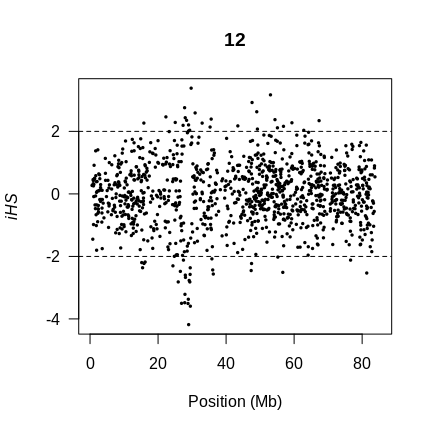# 2 Data input

The package rehh requires as input:

• a haplotype data file (see section 2.1).

and, if the haplotype data file is neither in variant call format nor in the format of ms output,

• a marker information file (see section 2.2).

Since R can automatically recognize and read compressed files with standard name extensions like .gz or .bzip2, this holds as well for the input files that can be used with this package.

## 2.1 Haplotype data file

Five haplotype input file formats are supported:

• a standard haplotype format. Each row represents a haplotype with marker genotype in columns as in the example file bta12_cgu.hap containing 280 haplotypes with 1424 SNPs each (see section 2.3.1). The first element of each row is taken as a haplotype identifier.
• a transposed format with haplotypes in columns and markers in rows as in the example file bta12_cgu.thap. This format is similar to the one produced by the phasing program SHAPEIT2 (O’Connell et al. 2014). This format assumes neither row nor column names and hence no haplotype identifiers can be specified (see section 2.3.2).
• the output file format from the phasing program fastPHASE (Scheet and Stephens 2006) as in the bta12_hapguess_switch.out example file. Note that this file format allows to include haplotypes from several populations (if the -u fastPHASE option was used) (see section 2.3.3).
• variant call format (vcf), comprising both haplotype and marker information. In order to read files in this format, the package vcfR or the packages data.table and R.utils (the latter is needed for compressed files) have to be installed (see section 2.3.4).
• The output of the simulation program ms (Hudson 2002) and its derivatives msHOT (Hellenthal and Stephens 2007), ms2 (Ewing and Hermisson 2010) and ms’ (Kelleher et al. 2016). In order to read these files, the package gap has to be installed (see section 2.3.5).

Alleles in standard or transposed haplotype format can be provided either coded (by integer numbers) or without coding (e.g. as nucleotides) (see section 2.3).

If alleles are not polarized, i.e. their ancestral/derived status is not known, some arbitrary assignation can be done either beforehand by the user or during input by the package; appropriate parameters must be set in subsequent functions (see section 7.6).

## 2.2 Marker information file

For haplotype data files in standard, transposed or fastphase format a marker information file has to be provided. The file should contain columns without header corresponding to:

1. marker name/identifier
2. name of the chromosome (or scaffold)
3. position on the chromosome (or scaffold), either physical (in bases) or genetic (e.g. in centiMorgans).

For a given chromosome, the markers must be ordered in the same way as in the haplotype data file.

If alleles in the haplotype file are not already provided in coded form (i.e. as positive integers), the package can (re)code them using two additional columns in the marker file:

1. ancestral allele
2. derived allele(s).

For instance, the provided file map.inp has five columns:

# show first 6 lines of file
cat(readLines("map.inp", n = 6), sep = "\n")
> F0100190 1 113642 T A
> F0100220 1 244699 C G
> F0100250 1 369419 G C
> F0100270 1 447278 A T
> F0100280 1 487654 T A
> F0100290 1 524507 C G

## 2.3 Loading data files: the function data2haplohh

The data2haplohh() function converts data files into an R object of class haplohh, forming the basis for subsequent analysis. The following options are available to recode alleles and/or to filter markers and haplotypes:

1. Allele coding options:

The parameter allele_coding has four options: three of them expect the user to provide a coding, while the fourth option overwrites any user defined coding.

The package accepts two different integer codings within the haplotype file which can be specified as "12" or "01", respectively:

“12” “01”
Missing value 0 NA or .
Ancestral allele 1 0
Derived allele 1 2 1
Derived allele 2 3 2

The latter one is also the coding used internally by our package.

If the alleles are given in an un-coded form (e.g. as nucleotides), the alleles can be coded for each marker separately with help of the marker information file. This option is activated by allele_coding = "map". If an allele of a marker is found in the fourth column of the marker information file, it is re-coded as 0 (ancestral) and if it is found in the fifth column it is re-coded as 1 (derived). The fifth column may contain several alleles, separated by commas, like in the variant call format. In this case, the internal coding extends to higher integers. If an allele present in the haplotype file is not found neither in the fourth nor the fifth column of the marker information file, it is counted as NA (missing value).

If the alleles are not polarized, i.e. it is unknown which allele is ancestral and which derived, the option "none" should be selected. The alleles will then be coded in alpha-numeric order for each marker; the first becomes 0, the second 1, etc. Missing values must be given as NA or ‘.’ while any other number, character or string will be counted as an allele. Options of subsequent analysis functions have to be set accordingly (see section 7.6).

2. Filtering options

2.1 Discard haplotypes with a high amount of missing data. If haplotypes contain missing data (presumably a rare case since most phasing programs allow imputing missing genotypes), it is possible to discard those with a fraction of less than min_perc_geno.hap of markers genotyped. Its default is NA, hence no restriction.

2.2. Discard markers with a high amount of missing data. It is possible to discard individual markers genotyped on a fraction of less than min_perc_geno.mrk of haplotypes. By default min_perc_geno.mrk = 100 meaning that only fully genotyped markers are retained. A value of NA or zero is not allowed since rehh cannot handle markers with no data.

2.3 Discard markers with a low Minor Allele Frequency. It is possible to discard markers with a Minor Allele Frequency (MAF) equal to or below min_maf. Its default is NA meaning no constraint. Setting this value to zero eliminates monomorphic markers. Note that since low-frequency alleles usually abound, any positive value may exclude a substantial fraction of the data.

The arguments min_perc_geno.hap, min_perc_geno.mrk and min_maf are evaluated in this order.

More details about input options can be found in the documentation using the command:

?data2haplohh

### 2.3.1 Example 1: reading haplotype file in standard format

The following command converts the haplotype input file bta12_cgu.hap (in “standard” haplotype format) and marker information input file map.inp to an haplohh object named hh. Because the map file contains information for markers mapping to multiple chromosomes we have to specify by chr.name = 12 that the haplotype input file is about chromosome 12. Alleles are given as nucleotides in the haplotype file and coded with help of the map file by setting allele_coding = "map".

hh <- data2haplohh(hap_file = "bta12_cgu.hap",
map_file = "map.inp",
chr.name = 12,
allele_coding = "map")
> * Reading input file(s) *
> Map info: 1424 markers declared for chromosome 12 .
> Haplotype input file in standard format assumed.
> Alleles are being recoded according to fourth and fifth column of map file.
> * Filtering data *
> Discard markers genotyped on less than 100 % of haplotypes.
> No marker discarded.
> Data consists of 280 haplotypes and 1424 markers.
> Number of mono-, bi-, multi-allelic markers:
> 1 2
> 27 1397

If no value is specified for chr.name and more than one chromosome is detected in the map file, an error is produced:

hh <- data2haplohh(hap_file = "bta12_cgu.hap",
map_file = "map.inp",
allele_coding = "map") 
> * Reading input file(s) *
> More than one chromosome name in file: map.inp .
> Here is a list of chromosome names found:
>  1 2 3 4 5 6 7 8 9 10 11 12 13 14 15 16 17 18 19 20 21 22 23 24 25 26 27 28 29
> Error: Please specify a chromosome name. Conversion stopped.

Furthermore, the following message is prompted if the number of markers for the chromosome in the info file does not correspond to the one in the haplotype file (for instance when a wrong chromosome is specified):

hh <- data2haplohh(hap_file = "bta12_cgu.hap",
map_file = "map.inp",
chr.name = 18,
allele_coding = "map")
> * Reading input file(s) *
> Map info: 1123 markers declared for chromosome 18 .
> Haplotype input file in standard format assumed.
> Error: The number of markers in the haplotype file (1424) is not equal
> to the number of markers in the map file (1123).
> Conversion stopped.

### 2.3.2 Example 2: reading haplotype file in transposed format (SHAPEIT2–like)

If the haplotype input file is in “transposed” format (like bta12_cgu.thap), the option haplotype.in.columns has to be set to TRUE while all other parameters remain unaffected with respect to example 1. This is the only format which is not recognized automatically, but has to be explicitly declared by the user.

hh <- data2haplohh(hap_file = "bta12_cgu.thap",
map_file = "map.inp",
chr.name = 12,
allele_coding = "map",
haplotype.in.columns = TRUE)
> * Reading input file(s) *
> Map info: 1424 markers declared for chromosome 12 .
> Haplotype input file in transposed format assumed.
> Alleles are being recoded according to fourth and fifth column of map file.
> * Filtering data *
> Discard markers genotyped on less than 100 % of haplotypes.
> No marker discarded.
> Data consists of 280 haplotypes and 1424 markers.
> Number of mono-, bi-, multi-allelic markers:
> 1 2
> 27 1397

### 2.3.3 Example 3: reading haplotype file in fastPHASE output format

In this example, we use the fastPHASE output bta12_hapguess_switch.out as haplotype file. This format is recognized automatically. As before, we need to set chr.name = 12. Because haplotypes originated here from several populations (the fastPHASE option -u was used), it is necessary to select the population of interest (in our case “CGU”) by its corresponding number in the file, hence popsel = 7.

hh <- data2haplohh(hap_file = "bta12_hapguess_switch.out",
map_file = "map.inp",
chr.name = 12,
popsel = 7,
allele_coding = "map")
> * Reading input file(s) *
> Map info: 1424 markers declared for chromosome 12 .
> Haplotype input file in fastPHASE format assumed.
> Haplotypes in the fastPHASE output file originate from 8 populations.
> Alleles are being recoded according to fourth and fifth column of map file.
> * Filtering data *
> Discard markers genotyped on less than 100 % of haplotypes.
> No marker discarded.
> Data consists of 280 haplotypes and 1424 markers.
> Number of mono-, bi-, multi-allelic markers:
> 1 2
> 27 1397

If no value is specified for the popsel argument and more than one population is detected in the fastPHASE output file, an error is produced and the available population numbers printed:

hh <- data2haplohh(hap_file = "bta12_hapguess_switch.out",
map_file = "map.inp",
chr.name = 12,
allele_coding = "map")
> * Reading input file(s) *
> Map info: 1424 markers declared for chromosome 12 .
> Haplotype input file in fastPHASE format assumed.
> Haplotypes in the fastPHASE output file originate from 8 populations.
> Error: Please specify by 'popsel' one of the following population numbers:
> 1 2 3 4 5 6 7 8
> Conversion stopped.

### 2.3.4 Example 4: reading vcf files

The function data2haplohh() checks automatically whether the specified haplotype input file is in variant call format (vcf). If this is the case, the parameters map_file and allele_coding are ignored. By default, the function tries to polarize (see section 7.6) the alleles of each marker using the ancestral allele, expected to be given by key AA of the INFO field.

Ancestral alleles are sometimes marked by upper case as “high confident” and by lower case as “low confident”. The default setting capitalize_AA = TRUE lifts this distinction before polarization.

If the AA key is absent, the option polarize_vcf should be set to FALSE and the allele coding of the vcf file is directly used as internal coding.

If there is data for more than one chromosome in the file, the chromosome of interest has to be specified by chr.name. Since always the whole file is read in, it is advisable to split large data sets into chromosome-specific files.

In order to process vcf files, the package vcfR or the package data.table (which in turn needs R.utils to read compressed files) have to be installed. The parameter vcf_reader has to be set to either "vcfR" or "data.table".

In the file bta12_cgu.vcf.gz the ancestral allele was set as reference and hence no further polarizing is necessary.

hh <- data2haplohh(hap_file = "bta12_cgu.vcf.gz",
polarize_vcf = FALSE,
vcf_reader = "data.table")
> * Reading input file(s) *
> Using package 'data.table' to read vcf.
> Extracting map information.
> Extracting haplotypes.
> Number of individuals which are
> Haploid Diploid Triploid, ... :
> 1 2
> 0 140
> * Filtering data *
> Discard markers genotyped on less than 100 % of haplotypes.
> No marker discarded.
> Data consists of 280 haplotypes and 1424 markers.
> Number of mono-, bi-, multi-allelic markers:
> 1 2
> 27 1397

### 2.3.5 Example 5: reading ms output

The function data2haplohh() automatically checks whether the haplotype file is in the output format of the simulation program ms (Hudson 2002). If this is the case, the parameters map_file and allele_coding are ignored. If the file contains several ‘runs’ (as referred to by the parameter nrep of ms), it is necessary to specify the number of the run in option chr.name. Note that always the whole file is read, so that it might be advisable to spread large simulations over separate files.

One argument of the data2haplohh function is specifically dedicated to ms output, although it works with other formats as well: ms gives chromosomal positions as fractions of the interval [0,1] and in order to obtain more realistic values, these positions can be multiplied by a factor, set by position_scaling_factor.

Note that ms output can contain multiple markers with the same (rounded) position, which rehh does not accept. In this case the numerical precision for chromosomal positions in the ms output should be increased (option -p of ms, option -oformat of msms).

Setting remove_multiple_markers to TRUE entails that from consecutive markers with the same position only the first one is retained and a warning containing the number of removed markers is printed. Note that this effectively transforms the “infinite sites model” used for simulations by ms into a “finite sites model”.

In order to read the ms format, the package gap has to be installed.

hh <- data2haplohh(hap_file = "ms.out",
chr.name = 2,
position_scaling_factor = 1000)
> * Reading input file(s) *
> Input file in 'ms' output format assumed.
>
> Extracting positions.
> Extracting haplotypes.
> * Filtering data *
> Discard markers genotyped on less than 100 % of haplotypes.
> No marker discarded.
> Data consists of 10 haplotypes and 39 markers.
> Number of mono-, bi-, multi-allelic markers:
> 1 2
> 0 39

## 2.4 Subset data

Sometimes it might be of interest to restrict the computation of statistics to subsets of haplotypes or markers. This can be done with help of the function subset() and arguments select.hap and select.mrk. After subsetting, the same filters are applied as during data input from files in order to ensure that no marker has exclusively missing values and, optionally, to exclude markers which are monomorphic within the subset by setting min_maf to zero.

For example, to restrict the output of ms (see section 2.3.5) to the first five haplotypes and filter out monomorphic sites, we apply

hh_subset = subset(hh, select.hap = 1:5, min_maf = 0)
> * Subset haplotypes *
> 5 haplotypes discarded.
> 5 haplotypes remaining.
> * Filtering data *
> Discard markers genotyped on less than 100 % of haplotypes.
> No marker discarded.
> Discard markers with Minor Allele Frequency equal to or below 0 .
> 30 markers discarded.
> 9 markers remaining.
> Data consists of 5 haplotypes and 9 markers.
> Number of mono-, bi-, multi-allelic markers:
> 1 2
> 0 9

while the following command omits the first marker:

hh_subset = subset(hh, select.mrk = -1)
> * Subset markers *
> 1 markers discarded.
> 38 markers remaining.
> * Filtering data *
> Discard markers genotyped on less than 100 % of haplotypes.
> No marker discarded.
> Data consists of 10 haplotypes and 38 markers.
> Number of mono-, bi-, multi-allelic markers:
> 1 2
> 0 38

# 3 Computing EHH, EHHS and their “integrals” iHH and iES

## 3.1 Definition and computation

### 3.1.1 The (allele-specific) Extended Haplotype Homozygosity (EHH)

For an allele $$a$$ of a focal marker $$s$$, sometimes referred to as a core allele, the Extended Haplotype Homozygosity (EHH) is defined as the probability that two randomly chosen chromosomes, carrying the core allele, are homozygous over a given surrounding chromosomal region (Sabeti et al. 2002). It is estimated from a sample by calculating the homozygosity of the chromosomal chunk between the focal marker and another marker $$t$$ by the formula $\begin{equation} \mathrm{EHH}_{s,t}^a=\frac{1}{n_{a}(n_a-1)}\sum\limits_{k=1}^{K^a_{s,t}}n_k(n_k-1) \tag{3.1} \end{equation}$ where $$n_a$$ represents the number of chromosomes carrying the core allele $$a$$, $$K^a_{s,t}$$ represents the number of different shared haplotypes and $$n_k$$ refers to the number of chromosomes pertaining to the $$k$$-th such shared haplotype. If there is no missing data, it holds that $$n_a=\sum\limits_{k=1}^{K^a_{s,t}}n_k$$.

In the case of unphased chromosomes from diploid individuals (see section 7.5) the extended haplotype homozygosity can be calculated as follows (Tang et al. 2007): we consider only chromosomes from individuals that are homozygous for the allele $$a$$ at the focal marker $$s$$ and estimate EHH at some marker $$t$$ by the fraction of individuals that are (still) homozygous over the entire chromosomal stretch between $$s$$ and $$t$$. Let $$I^a_{s,t}$$ denote the number of individuals that are homozygous from marker $$s$$ til marker $$t$$. $\begin{equation} \mathrm{EHH}_{s,t}^a=\frac{I_{s,t}^a}{I_{s,s}^a}\;. \tag{3.2} \end{equation}$

EHH is usually computed only for a region it surpasses a given threshold (e.g., $$EHH > 0.05$$).

### 3.1.2 The integrated EHH (iHH)

By definition, EHH starts at 1 and decays to 0 with increasing distance of t from the focal marker s. For a given core allele, the integrated EHH (iHH) is defined as the area under the EHH curve which, in turn, is defined by the EHH values and associated chromosomal positions (Voight et al. 2006). The integral is computed with a simple numerical method, called the trapezoidal rule.

Note that, technical details aside, the iHH value is nothing else than the average length of shared haplotypes.

### 3.1.3 The (site-specific) Extended Haplotype Homozygosity (EHHS)

An extended haplotype homozygosity can be defined as well without regard to core alleles. In this case, the quantity is aimed to reflect the probability that any two randomly chosen chromosomes from a population are homozygous over a given surrounding chromosomal region of a focal marker. In contrast to the allele-specific EHH defined in the previous section, the chromosomes are not required to carry a specific allele at the focal marker. We adopt the naming by (Tang et al. 2007) as site–specific EHH, abbreviated by EHHS. Note, however that this quantity is sometimes referred to as EHH, too, and there is no agreed notation in the literature.

EHHS was used in genome scans in two versions: un-normalized by (Sabeti et al. 2007) and normalized by (Tang et al. 2007).

In line with (Sabeti et al. 2007) we define $\begin{equation} \tag{3.3} \mathrm{EHHS}_{s,t}=\frac{1}{n_s(n_s-1)}\sum\limits_{k=1}^{K_{s,t}}n_k(n_k-1) \end{equation}$ where we re-use notation from above and let $$n_s$$ refer to the number of chromosomes at marker $$s$$. If there are no missing values at that marker, this is simply the number of chromosomes in the sample.

(Tang et al. 2007) proposed an apparently different estimator for the normalized EHHS, which we abbreviate to nEHHS, namely $\begin{equation} \tag{3.4} \mathrm{nEHHS}_{s,t}=\frac{1-h_{s,t}}{1-h_s} \end{equation}$ where:

1. $$h_s=\frac{n_s}{n_s-1}\left(1-\frac{1}{n_s^2}\left(\sum\limits_{k=1}^{K_{s,s}}n_k^2 \right )\right)$$ is an estimator of the focal marker heterozygosity. In the bi-allelic case we can write this as $$h_s=\frac{n_s}{n_s-1}\left(1-\frac{1}{n_s^2}\left(n_{a1}^2+n_{a2}^2\right) \right)$$ with $$a1$$ and $$a2$$ referring to the numbers of the two alleles at marker $$s$$ ($$n_{a1}+n_{a2}=n_s$$).

2. $$h_{s,t}=\frac{n_s}{n_s-1}\left(1-\frac{1}{n_s^2}\left(\sum\limits_{k=1}^{K_{s,t}}n_k^2 \right )\right)$$ is an estimator of haplotype heterozygosity in the chromosomal region extending from marker $$s$$ to marker $$t$$.

However both definitions are in fact equivalent, because it holds $$\mathrm{EHHS}_{s,t}=1-h_{s,t}$$ and hence $\begin{equation*} \mathrm{nEHHS}_{s,t}=\frac{\mathrm{EHHS}_{s,t}}{\mathrm{EHHS}_{s,s}}\;. \end{equation*}$ Thus $$\mathrm{nEHHS}_{s,t}$$ is just normalized in order to yield 1 at the focal marker $$s$$. Note that the normalization factor depends on the frequency of the alleles at the focal marker and consequently is not necessarily the same for different focal markers.

Furthermore, we note that EHHS and EHH are related by $\begin{equation*} \mathrm{EHHS}_{s,t}=\frac{n_{a1}(n_{a1}-1)}{n_s(n_s-1)}\mathrm{EHH}_{s,t}^{a1}+\frac{n_{a2}(n_{a2}-1)}{n_s(n_s-1)}\mathrm{EHH}^{a2}_{s,t}\;, \end{equation*}$ where for the sake of simplicity we assume that the focal marker has only two alleles $$a1$$ and $$a2$$. EHHS might hence be viewed as a linear combination of the EHH’s of the focal alleles, weighted by roughly the square of the focal allele frequencies.

In the case of unphased chromosomes from diploid individuals (see section 7.5) EHHS can be calculated like EHH in Equation (3.2), just without reference to core alleles: $\begin{equation} \mathrm{EHHS}_{s,t}=\frac{I_{s,t}}{I_{s,s}}\;. \tag{3.5} \end{equation}$ Note that defined this way, EHHS is always 1 at the focal marker. Hence there is no distinction between $$\mathrm{EHHS}$$ and $$\mathrm{nEHHS}$$.

Again, unphased EHHS can be related to unphased EHH by $\begin{equation} \mathrm{EHHS}_{s,t}=\frac{I_{s,t}}{I_{s,s}}=\frac{I_{s,t}^{a1}+I_{s,t}^{a2}}{I_{s,s}^{a1}+I_{s,s}^{a2}}=\frac{I_{s,s}^{a1}}{I_{s,s}^{a1}+I_{s,s}^{a2}}\mathrm{EHH}_{s,t}^{a1}+\frac{I_{s,s}^{a2}}{I_{s,s}^{a1}+I_{s,s}^{a2}}\mathrm{EHH}_{s,t}^{a2}\; \end{equation}$ where for the sake of simplicity we assumed a bi-allelic focal marker with alleles $$a1$$ and $$a2$$.

As with EHH, the EHHS is usually computed only for the region where its value surpasses a given threshold (e.g., $$EHHS > 0.05$$).

### 3.1.4 The integrated EHHS (iES)

Like EHH, EHHS has its maximum at the focal marker and decays to 0 with increasing distance from the focal marker. For a given focal marker, analogously to iHH, iES is defined as the integrated EHHS (Tang et al. 2007). Depending on whether EHHS or nEHHS is integrated, we yield iES and inES respectively. As with iHH, the numerical integration uses the trapezoidal rule.

Note that, technical details aside, the iES and inES values represent the average length of shared haplotypes. The length of shared haplotypes with different core alleles yields zero and these are included in the former but not the latter.

## 3.2 The function calc_ehh()

The function calc_ehh() computes EHH for all alleles of a focal marker $$s$$ relative to markers $$t$$ upstream and downstream. For each allele the corresponding integral iHH of the EHH curve is calculated as well.

Three options can be specified to constrain the computation of EHH:

• limehh sets a threshold below which further calculation of EHH is stopped. Its default value is 0.05. Note that lowering this cut-off might actually decrease the power to detect selective events since under neutrality a tiny fraction of sequences has very long shared haplotypes which, if not capped, confound the signal of selection (Klassmann and Gautier 2020).

• limhaplo defines the smallest acceptable number of evaluated chromosomes and has a default (and mimimum) value of 2. This parameter might be increased if missing values are suspected to be non-randomly distributed leading to a biased drop-out of evaluated chromosomes.

• limhomohaplo sets a minimum number of homozygous chromosomes below which calculation of EHH is stopped (or not even started). Its default (and minimum) value is 2. This number should be increased to 4 for small samples of unphased haplotypes in order to limit the influence of a single shared haplotype (see section 7.5.)

Several parameters influence the IHH values (the integral over EHH):

• by default, when the calculation of EHH reaches the border of the chromosome, i.e. the first or last marker, or encounters a large gap (see below), the integral iHH is discarded in order to avoid possible boundary effects. In order to retain the integrals set discard_integration_at_border to FALSE.

• to account for gaps between consecutive markers, two parameters can be set (see also section 7.2)

• gaps greater than scalegap are re-scaled (capped) to that size.
• integration is stopped/discarded if a gap greater than maxgap is encountered.
• integration is performed by default over the area between the graph defined by the EHH values and the horizontal line y = limehh. If numerical agreement with the program Hapbin is wanted, this area should be extended to the x-axis by setting lower_y_bound to zero.

• by default, the EHH curve is defined by linearly interpolating EHH values between consecutive markers, yielding a continuous curve. However, in particular for full re-sequencing data, it is more accurate to let this function decrease step-wise at each marker by setting interpolate to FALSE (although the difference is likely to be minor).

The option polarized, TRUE by default, in this function merely affects the order and labeling of alleles.

The parameter phased can be toggled to FALSE in order to calculate EHH by the formula for unphased data (see section 3.1.1 and 7.5).

The option include_zero_values concerns only the output. If FALSE (by default), the output contains only EHH values for markers up to the specified cut-offs. If the parameter is toggled to TRUE, then values for all markers are returned, the remaining ones being zero.

Details are available in the R documentation by using the command:

?calc_ehh

In the following example, EHH is computed around the SNP F1205400. This SNP is associated with a strong signal of selection (using both iHS and Rsb statistics) and is located closely (<5kb) to a strong candidate gene involved in horn development (Gautier and Naves 2011).

Note that the R object haplohh_cgu_bta12 was generated using the data2haplohh() function with the example input files (see section 2.3.1).

#example haplohh object (280 haplotypes, 1424 SNPs) see ?haplohh_cgu_bta12 for details
data(haplohh_cgu_bta12)
#computing EHH statistics for the focal SNP with name "F1205400"
#which displays a strong signal of selection
res <- calc_ehh(haplohh_cgu_bta12,
mrk = "F1205400",
include_nhaplo = TRUE)

The output contained in res is a list with four elements:

1. mrk.name: the name/identifier of the focal marker.
2. freq: a vector containing the frequencies of all alleles of the focal marker.
3. ehh: a data frame containing the EHH values for each allele of the focal marker. Setting the parameter include_nhap to TRUE adds columns which show how many chromosomes contributed at each position to the calculation of EHH.
4. ihh: a vector with iHH for each allele, i.e. the integrals over the EHH curves defined by the EHH values.
res$mrk.name >  "F1205400" res$freq
>    FREQ_A    FREQ_D
> 0.3035714 0.6964286
res$ehh > POSITION EHH_A EHH_D NHAPLO_A NHAPLO_D > F1204760 25629699 0.00000000 0.05175786 0 195 > F1204770 25680969 0.00000000 0.05175786 0 195 > F1204780 25727883 0.00000000 0.05207507 0 195 > F1204790 25769786 0.00000000 0.05239228 0 195 > F1204800 25884193 0.00000000 0.05239228 0 195 > F1204810 25932431 0.00000000 0.05249802 0 195 > F1204840 26132031 0.00000000 0.05884219 0 195 > F1204850 26189286 0.00000000 0.05884219 0 195 > F1204870 26255986 0.00000000 0.05884219 0 195 > F1204880 26313792 0.00000000 0.05889506 0 195 > F1204900 26479781 0.00000000 0.05926513 0 195 > F1204910 26507987 0.00000000 0.05926513 0 195 > F1204920 26526646 0.00000000 0.05926513 0 195 > F1204930 26558947 0.00000000 0.06650806 0 195 > F1204940 26599290 0.00000000 0.08675654 0 195 > F1204950 26683271 0.00000000 0.08675654 0 195 > F1204960 26727974 0.00000000 0.11134021 0 195 > F1204970 26779948 0.00000000 0.12513878 0 195 > F1204980 26800201 0.00000000 0.12778218 0 195 > F1204990 26854850 0.00000000 0.13068993 0 195 > F1205000 26902773 0.00000000 0.13967750 0 195 > F1205010 26952729 0.00000000 0.25424266 0 195 > F1205020 26997449 0.00000000 0.37520486 0 195 > F1205030 27031612 0.00000000 0.42008988 0 195 > F1205040 27058533 0.00000000 0.42024848 0 195 > F1205050 27097980 0.00000000 0.47269363 0 195 > F1205070 27200291 0.00000000 0.47269363 0 195 > F1205080 27356111 0.00000000 0.51578113 0 195 > F1205090 27378189 0.00000000 0.52307692 0 195 > F1205100 27465862 0.00000000 0.52307692 0 195 > F1205110 27501592 0.00000000 0.52312979 0 195 > F1205120 27525360 0.00000000 0.53782712 0 195 > F1205130 27588853 0.00000000 0.53782712 0 195 > F1205150 27735666 0.00000000 0.53782712 0 195 > F1205160 27804138 0.00000000 0.54290246 0 195 > F1205170 27833642 0.00000000 0.56674597 0 195 > F1205180 27907564 0.00000000 0.78752313 0 195 > F1205190 27985596 0.00000000 0.80586836 0 195 > F1205200 28014498 0.00000000 0.80602696 0 195 > F1205210 28054852 0.00000000 0.81527888 0 195 > F1205230 28266218 0.00000000 0.85249802 0 195 > F1205240 28296968 0.00000000 0.85249802 0 195 > F1205250 28386447 0.00000000 0.85249802 85 195 > F1205260 28426219 0.05854342 0.90023791 85 195 > F1205270 28562210 0.06694678 0.90023791 85 195 > F1205280 28606777 0.06750700 0.90023791 85 195 > F1205290 28633388 0.08767507 0.91979910 85 195 > F1205300 28689805 0.13137255 0.95945017 85 195 > F1205310 28721292 0.14649860 0.95945017 85 195 > F1205320 28753422 0.16246499 0.95945017 85 195 > F1205330 28786001 0.16246499 0.95945017 85 195 > F1205340 28810078 0.16246499 0.95945017 85 195 > F1205360 28896769 0.16610644 0.98974359 85 195 > F1205370 28925117 0.19103641 1.00000000 85 195 > F1205380 28947722 0.27647059 1.00000000 85 195 > F1205390 28967990 0.55294118 1.00000000 85 195 > F1205400 28993983 1.00000000 1.00000000 85 195 > F1205420 29101326 0.88795518 1.00000000 85 195 > F1205440 29147373 0.64229692 1.00000000 85 195 > F1205450 29197279 0.59551821 1.00000000 85 195 > F1205460 29295437 0.29691877 0.98974359 85 195 > F1205470 29337933 0.20364146 0.96944224 85 195 > F1205480 29424210 0.14145658 0.91044145 85 195 > F1205490 29456634 0.08207283 0.90066085 85 195 > F1205500 29492629 0.07759104 0.67512556 85 195 > F1205530 29576146 0.07759104 0.67512556 85 195 > F1205540 29607285 0.06218487 0.66545070 85 195 > F1205550 29642190 0.00000000 0.66180280 85 195 > F1205560 29727300 0.00000000 0.48183981 0 195 > F1205570 29885809 0.00000000 0.24160719 0 195 > F1205580 29933214 0.00000000 0.17055247 0 195 > F1205590 29953532 0.00000000 0.11937616 0 195 > F1205600 30060316 0.00000000 0.11937616 0 195 > F1205610 30148780 0.00000000 0.10134814 0 195 > F1205620 30176268 0.00000000 0.10134814 0 195 > F1205640 30241555 0.00000000 0.06111552 0 195 > F1205650 30270049 0.00000000 0.05667460 0 195 > F1205660 30472702 0.00000000 0.05551150 0 195 > F1205680 30532042 0.00000000 0.05551150 0 195 res$ihh
>     IHH_A     IHH_D
>  284429.9 2057107.4

The EHH values can be plotted by setting the output of calc_ehh() into the function plot() to yield Figure 3.1:

plot(res)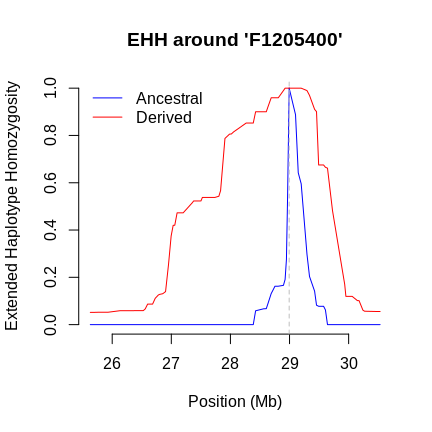Figure 3.1: Graphical output of the plot.ehh() function

For comparison, we might ignore that haplotypes have been phased and set the option phased to FALSE. Figure 3.2 shows that for this particular marker the differences are rather small:

plot(calc_ehh(haplohh_cgu_bta12,
mrk = "F1205400",
phased = FALSE),
xlim = c(2.55E7, 3.05E7))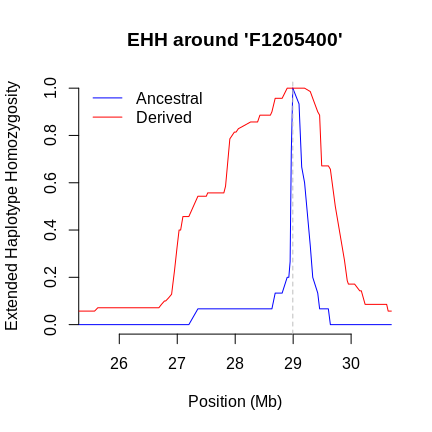Figure 3.2: Graphical output of the plot.ehh() function

## 3.3 The function calc_ehhs()

The calc_ehhs() function computes EHHS and normalized EHHS around the focal marker $$s$$ relative to another marker $$t$$. This function also computes the corresponding integrals iES and inES respectively. The options are identical to those of the function calc_ehh (see previous section), except that polarized is absent, because variant ancestry status does not figure in the formulas. Details are available by the command:

?calc_ehhs

In the following example, the EHHS statistics are computed around the SNP F1205400.

data(haplohh_cgu_bta12)
res <- calc_ehhs(haplohh_cgu_bta12,
mrk = "F1205400",
include_nhaplo = TRUE)

The output is similar to that of calc_ehh(), except that there are no alleles to be distinguished, but instead the wether EHHS is normalized or not. A list with four elements is obtained:

1. mrk.name: the name/identifier of the focal marker.
2. ehhs: a data frame with EHHS and nEHHS values along the chromosome around the focal marker. Optionally, the column NHAPLO can be included to show how many chromosomes were evaluated at each marker.
3. IES: iES, i.e. the integral over the EHHS curve.
4. INES: inES, i.e. the integral over the nEHHS curve.
res$mrk.name >  "F1205400" res$ehhs 
>          POSITION       EHHS      NEHHS NHAPLO
> F1204840 26132031 0.00000000 0.05132310    280
> F1204850 26189286 0.00000000 0.05132310    280
> F1204870 26255986 0.00000000 0.05132310    280
> F1204880 26313792 0.00000000 0.05136758    280
> F1204900 26479781 0.00000000 0.05167890    280
> F1204910 26507987 0.00000000 0.05167890    280
> F1204920 26526646 0.00000000 0.05167890    280
> F1204930 26558947 0.00000000 0.05777185    280
> F1204940 26599290 0.00000000 0.07480543    280
> F1204950 26683271 0.00000000 0.07480543    280
> F1204960 26727974 0.05499232 0.09553035    280
> F1204970 26779948 0.06190476 0.10753836    280
> F1204980 26800201 0.06318484 0.10976206    280
> F1204990 26854850 0.06464414 0.11229709    280
> F1205000 26902773 0.06899642 0.11985768    280
> F1205010 26952729 0.12450077 0.21627752    280
> F1205020 26997449 0.18312852 0.31812319    280
> F1205030 27031612 0.20486431 0.35588170    280
> F1205040 27058533 0.20509473 0.35628197    280
> F1205050 27097980 0.23049155 0.40040027    280
> F1205070 27200291 0.23049155 0.40040027    280
> F1205080 27356111 0.25163850 0.43713587    280
> F1205090 27378189 0.25517153 0.44327329    280
> F1205100 27465862 0.25517153 0.44327329    280
> F1205110 27501592 0.25519713 0.44331777    280
> F1205120 27525360 0.26231439 0.45568157    280
> F1205130 27588853 0.26233999 0.45572604    280
> F1205150 27735666 0.26233999 0.45572604    280
> F1205160 27804138 0.26490015 0.46017345    280
> F1205170 27833642 0.27667691 0.48063153    280
> F1205180 27907564 0.38374296 0.66662219    280
> F1205190 27985596 0.39272913 0.68223260    280
> F1205200 28014498 0.39298515 0.68267734    280
> F1205210 28054852 0.39782386 0.69108294    280
> F1205230 28266218 0.41610343 0.72283745    280
> F1205240 28296968 0.41610343 0.72283745    280
> F1205250 28386447 0.41610343 0.72283745    280
> F1205260 28426219 0.44129544 0.76659996    280
> F1205270 28562210 0.44206349 0.76793418    280
> F1205280 28606777 0.44211470 0.76802313    280
> F1205290 28633388 0.45343062 0.78768068    280
> F1205300 28689805 0.47662570 0.82797421    280
> F1205310 28721292 0.47800819 0.83037581    280
> F1205320 28753422 0.47946749 0.83291083    280
> F1205330 28786001 0.47946749 0.83291083    280
> F1205340 28810078 0.47946749 0.83291083    280
> F1205360 28896769 0.49447005 0.85897265    280
> F1205370 28925117 0.50171531 0.87155882    280
> F1205380 28947722 0.50952381 0.88512342    280
> F1205390 28967990 0.53479263 0.92901935    280
> F1205400 28993983 0.57565284 1.00000000    280
> F1205420 29101326 0.56541219 0.98221036    280
> F1205440 29147373 0.54295955 0.94320658    280
> F1205450 29197279 0.53868408 0.93577941    280
> F1205460 29295437 0.50642601 0.87974205    280
> F1205470 29337933 0.48806964 0.84785412    280
> F1205480 29424210 0.45381464 0.78834779    280
> F1205490 29456634 0.44365079 0.77069157    280
> F1205500 29492629 0.33402458 0.58025350    280
> F1205530 29576146 0.33402458 0.58025350    280
> F1205540 29607285 0.32793139 0.56966867    280
> F1205550 29642190 0.32501280 0.56459862    280
> F1205560 29727300 0.23663594 0.41107405    280
> F1205570 29885809 0.12012289 0.20867245    280
> F1205580 29933214 0.08553507 0.14858795    280
> F1205590 29953532 0.06075269 0.10553702    280
> F1205600 30060316 0.06075269 0.10553702    280
> F1205610 30148780 0.05192012 0.09019346    280
> F1205620 30176268 0.05192012 0.09019346    280
> F1205640 30241555 0.00000000 0.05532577    280
> F1205650 30270049 0.00000000 0.05154547    280
> F1205660 30472702 0.00000000 0.05012230    280
> F1205680 30532042 0.00000000 0.05012230    280
res$IES >  936407.6 res$INES
>  1760565

Figure 3.3 can be obtained by setting the output of calc_ehhs into the function plot.ehhs():

plot(res)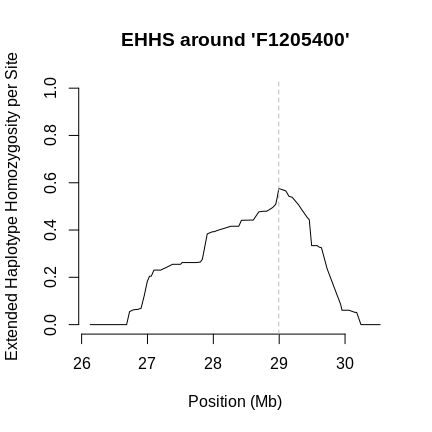Figure 3.3: Graphical output of the plot.ehhs() function

By default, the un-normalized EHHS values are plotted. This can be toggled by setting nehhs = TRUE. As can be seen in Figure 3.4, the values are scaled to yield 1 at the focal marker.

plot(res, nehhs = TRUE)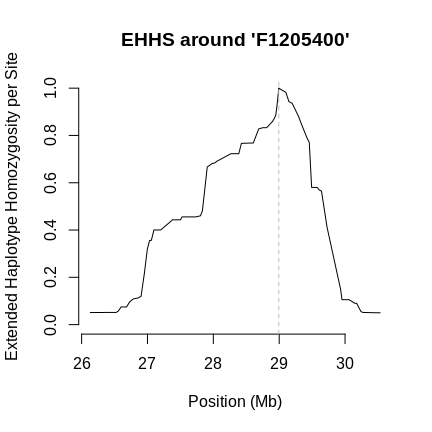Figure 3.4: Graphical output of the plot.ehhs() function

The parameter phased of the function calc_ehhs() can be toggled to FALSE yielding Figure 3.5. As described above, for unphased data the EHHS values are always normalized. For this particular marker we get a similar picture as for phased nEHHS.

plot(calc_ehhs(haplohh_cgu_bta12,
mrk = "F1205400",
phased = FALSE))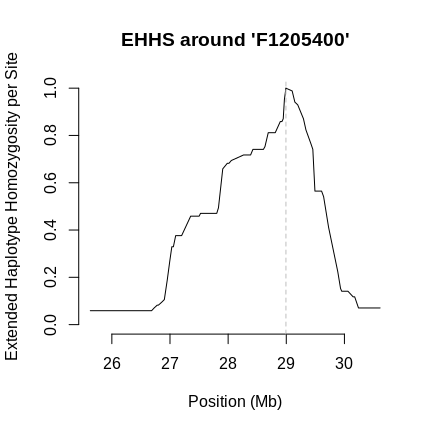Figure 3.5: Graphical output of the plot.ehhs() function

## 3.4 The function scan_hh()

While the functions calc_ehh() and calc_ehhs() return the integrated statistics iHH and iES for a particular marker, the function scan_hh() calculates these values for all markers in the haplohh object. However, in contrast to calc_ehh() which computes an iHH value for each allele of a possibly multi-allelic focal marker, scan_hh() calculates only two iHH values, namely (by default) for the ancestral allele and the derived allele with highest frequency. In case of unpolarized markers (see section 7.6), the option polarized should be set to FALSE, causing the function to use major and minor (most frequent and second most frequent) alleles instead.

The remainder of options are essentially the same as for the functions calc_ehh() and calc_ehhs() (see section 3.1.1). Details are given in the documentation:

?scan_hh

As an example, in order to scan the haplohh_cgu_bta12 object (containing data on 1424 SNPs for 280 haplotypes), one may use the following command:

data(haplohh_cgu_bta12)
scan <- scan_hh(haplohh_cgu_bta12)

The resulting object scan is a data frame with 1424 rows (the number of markers declared in the haplohh object) and 10 columns:

1. chromosome name
2. position in the chromosome
3. sample frequency of the ancestral / major allele
4. sample frequency of the second-most frequent remaining allele
5. number of evaluated haplotypes at the focal marker for the ancestral / major allele
6. number of evaluated haplotypes at the focal marker for the second-most frequent remaining allele
7. iHH of the ancestral / major allele
8. iHH of the second-most frequent remaining allele
9. iES
10. inES

Note that while for phased data the number of evaluated haplotypes of a core allele corresponds to its frequency in the sample, in case of unphased data the evaluation is restricted to haplotypes of homozygous individuals.

The following command prints a chunk of the scan containing the marker F1205400:

scan[453:459,]
>          CHR POSITION     FREQ_A    FREQ_D NHAPLO_A NHAPLO_D     IHH_A
> F1205370  12 28925117 0.06071429 0.9392857       17      263  765720.1
> F1205380  12 28947722 0.82500000 0.1750000      231       49 1477031.7
> F1205390  12 28967990 0.90000000 0.1000000      252       28 1211909.5
> F1205400  12 28993983 0.30357143 0.6964286       85      195  284429.9
> F1205420  12 29101326 0.01785714 0.9821429        5      275  269322.2
> F1205440  12 29147373 0.05714286 0.9428571       16      264  336600.7
> F1205450  12 29197279 0.04285714 0.9571429       12      268  528732.6
>              IHH_D       IES    INES
> F1205370 1121814.8  971728.6 1119699
> F1205380  454891.9  966416.3 1433143
> F1205390  574301.6  954903.3 1204234
> F1205400 2057107.4  936407.6 1760565
> F1205420 1086530.3 1041943.5 1086279
> F1205440 1185898.6 1036274.2 1182946
> F1205450 1149951.0 1039850.3 1148696

Note that scan_hh() is more efficient than calc_ehh() and calc_ehhs() applied consecutively for each marker as can be seen by running the two code snippets below:

# perform scan using scan_hh
system.time(scan <- scan_hh(haplohh_cgu_bta12))
>    user  system elapsed
>   0.865   0.000   0.870
# perform scan applying calc_ehh and calc_ehhs to each marker
slow_scan_hh <- function(haplohh) {
# create empty vectors of size nmrk
IHH_A <- IHH_D <- IES <- INES <- vector("numeric", nmrk(haplohh))
# invoke calc_ehh and calc_ehhs for each marker
for (i in 1:nmrk(haplohh)) {
res <- calc_ehh(haplohh, mrk = i)
IHH_A[i] <- res$ihh["IHH_A"] IHH_D[i] <- res$ihh["IHH_D"]
res <- calc_ehhs(haplohh, mrk = i)
IES[i] <- res$IES INES[i] <- res$INES
}
# create data frame (the return value of this function)
data.frame(IHH_A = IHH_A,
IHH_D = IHH_D,
IES = IES,
INES = INES)
}
system.time(slow_scan <- slow_scan_hh(haplohh_cgu_bta12))
>    user  system elapsed
>   5.237   0.000   5.287

Comparing columns shows that the computed values are identical:

identical(slow_scan[, "IHH_A"], scan[, "IHH_A"])
>  TRUE
identical(slow_scan[, "IHH_D"], scan[, "IHH_D"])
>  TRUE
identical(slow_scan[, "IES"], scan[, "IES"])
>  TRUE
identical(slow_scan[, "INES"], scan[, "INES"])
>  TRUE

# 4 Computing iHS, Rsb and XP-EHH

## 4.1 The iHS within-population statistic

### 4.1.1 Definition

The abbreviation iHS refers to “integrated haplotype homozygosity score”. Let uniHS represent the un-standardized log-ratio of ancestral iHH$$^A$$ to derived iHH$$^D$$ of a certain focal marker $$s$$: $\mathrm{uniHS}(s)=\ln\left(\frac{\mathrm{iHH}^A(s)}{\mathrm{iHH}^D(s)}\right)$ Following (Voight et al. 2006) we perform a standardization by setting: $\mathrm{iHS}(s)=\frac{\mathrm{uniHS}(s) - \mathrm{mean}(\mathrm{uniHS}|p_s)}{\mathrm{sd}(\mathrm{uniHS}|p_s)}$ where $$\mathrm{mean}(\mathrm{uniHS}|p_s)$$ and $$\mathrm{sd}(\mathrm{uniHS}|p_s)$$ represent the mean and standard deviation of uniHS restricted to those markers with a similar derived allele frequency as observed at the focal marker ($$p_s$$). In practice, the markers are binned so that each bin contains not a single frequency but a small range of frequencies to yield enough markers for reliable estimates of mean and standard deviation.

iHS is constructed to have an approximately standard Gaussian distribution and to be comparable across markers regardless of their underlying allele frequencies.

For a practical characterization of “outliers” we stretch statistical notation and associate a p-value to iHS (Gautier and Naves 2011) by: $p_\mathrm{iHS}=-\log_{10}\left(2\Phi\left(-|\mathrm{iHS}|\right)\right)$ where $$\Phi\left(x\right)$$ represents the Gaussian cumulative distribution function.

Assuming most of genotyped markers behave neutrally and the distribution of iHS values for neutral markers follows indeed a standard Gaussian distribution, we might interpret $$p_\text{iHS}$$ as a two-sided p-value (on a $$-\log_{10}$$ scale) of a test on the null hypothesis of no selection.

Alternatively, a one-sided p-value (in a $$-\log_{10}$$ scale) might be defined (Gautier and Naves 2011) as: $\begin{equation*} p^\text{left}_\text{iHS}=-\log_{10}\left(\Phi\left(\text{iHS}\right)\right) \end{equation*}$ allowing the identification of those sites where the derived allele displays a significantly higher extended haplotype homozygosity than the ancestral allele or $\begin{equation*} p^\text{right}_\text{iHS}=-\log_{10}\left(1-\Phi\left(\text{iHS}\right)\right) \end{equation*}$ for the opposite case.

Note that this procedure is controversial, because we identify the empirical distribution with the distribution under the null hypothesis of neutrality. This is an approximation at best and only warranted when it can be assumed that there are so few selected sites that their influence on the overall shape of the distribution can be neglected.

In case of unpolarized alleles, the uniHS is taken as the ratio of iHH from minor to major allele. Since derived allele frequency cannot be accounted for, no binning should be performed. The resulting standardized iHS cannot be expected to follow a normal distribution and p-values as defined above are not meaningful.

### 4.1.2 The function ihh2ihs()

The ihh2ihs() function computes iHS using a data frame containing the iHH values for ancestral and derived (resp. major and minor) alleles as obtained by the scan_hh() function (see section 3.4). The argument min_maf allows to exclude focal markers according to their minor allele frequency (by default min_maf=0.05). The argument freqbin controls the size (or number) of the allele frequency bins used to perform standardization (see section 4.1.1). More precisely, allele frequency bins are built from min_maf to 1-min_maf in steps of size freqbin (by default freqbin=0.025). If an integer of 1 or greater is specified, a corresponding number of equally spaced bins is created. If freqbin is set to 0, standardization is performed considering each observed frequency as a discrete frequency class, which is useful when there are only a few distinct haplotypes.

For unphased data, iHH is calculated using only haplotypes of individuals which are homozygous at the focal marker. This number can be considerably lower than the absolute allele frequency. Hence, in addition to min_maf, the option min_nhaplo (default NA) should be used to reduce statistical noise arising from too few evaluated haplotypes.

Optionally, the allele frequencies of the input data frame can be included into the output by setting include_freq to TRUE.

A p-value is calculated for standardized iHS values. By default, it is two-sided, but a side can be chosen by setting argument p.side to "left" or "right".

As a typical workflow for performing a whole genome scan one might run scan_hh() on haplotype data from each chromosome and concatenate the resulting data frames before standardization. In the following example, we assume that the haplotype files are named as hap_chr_i.cgu with $$i=1,...,29$$ and the marker information file is named map.inp. The data frame wgscan contains the $$iHH^A$$ and $$iHH^D$$ values for the whole genome and serves as input for the ihh$ihs() function which calculates iHS, i.e. the standardized log ratio of the two $$iHH$$ values, for each marker. ## demo code - no data files for all chromosomes provided for(i in 1:29) { # haplotype file name for each chromosome hap_file = paste("hap_chr_", i, ".cgu", sep = "") # create internal representation hh <- data2haplohh(hap_file = hap_file, map_file = "map.inp", chr.name = i, allele_coding = "map") # perform scan on a single chromosome (calculate iHH values) scan <- scan_hh(hh) # concatenate chromosome-wise data frames to # a data frame for the whole genome # (more efficient ways certainly exist...) if (i == 1) { wgscan <- scan } else { wgscan <- rbind(wgscan, scan) } } # calculate genome-wide iHS values wgscan.ihs <- ihh2ihs(wgscan) For illustration, the object wgscan.cgu contains the calculated and concatenated $$iHH$$ values of a whole genome scan on population CGU (Gautier and Naves 2011): library(rehh.data) data(wgscan.cgu) wgscan.ihs.cgu <- ihh2ihs(wgscan.cgu) > Discard focal markers with Minor Allele Frequency equal to or below 0.05 . > 6890 markers discarded. > 37167 markers remaining. The resulting object wgscan.ihs.cgu is a list with two elements: 1. ihs: a data frame with iHS and corresponding p-value $$p_\mathrm{iHS}$$ (p-value in a $$-\log_{10}$$ scale assuming the iHS are normally distributed under the neutral hypothesis). The first rows are displayed below: head(wgscan.ihs.cgu$ihs)
>          CHR POSITION        IHS LOGPVALUE
> F0100190   1   113642 -0.5582992 0.2390952
> F0100220   1   244699  0.2723337 0.1049282
> F0100250   1   369419  0.4810736 0.2003396
> F0100270   1   447278  1.0618710 0.5401640
> F0100280   1   487654  0.8184060 0.3839181
> F0100290   1   524507 -0.3897024 0.1569189
1. frequency.class: a data frame summarizing the derived allele frequency bins used for standardization and mean and standard deviation of the un-standardized values. The first rows are shown below:
head(wgscan.ihs.cgu$frequency.class) > N_MRK MEAN_UNIHS SD_UNIHS LOWER_QT UPPER_QT > [0.05,0.075) 1090 -0.7117920 0.6106032 -2.030274 0.2952986 > [0.075,0.1) 907 -0.5788939 0.5703576 -1.833828 0.3448781 > [0.1,0.125) 787 -0.4572926 0.4875843 -1.563436 0.3611656 > [0.125,0.15) 837 -0.3992265 0.4542230 -1.403821 0.3629034 > [0.15,0.175) 824 -0.3484786 0.4341126 -1.253109 0.4850874 > [0.175,0.2) 886 -0.2808167 0.4621221 -1.297452 0.5788857 ## 4.2 The Rsb pairwise population statistic ### 4.2.1 Definition The abbreviation Rsb stands for “ratio of EHHS between populations”. For a given marker $$s$$, let $\mathrm{unRsb}(s)=\ln\left(\frac{\mathrm{inES}_\text{pop1}(s)}{\mathrm{inES}_\text{pop2}(s)}\right)$ represent the log-ratio of the inES values computed in two populations $$pop1$$ and $$pop2$$ (see section 3.1.4). The Rsb for marker $$s$$ is defined as the standardized unRsb (Tang et al. 2007): $\begin{equation*} \mathrm{Rsb(s)}=\frac{\mathrm{unRsb}(s) - \text{median}({\mathrm{unRsb}})}{\mathrm {sd}({\mathrm{unRsb})}} \end{equation*}$ where $$\text{median}(\mathrm{unRsb})$$ and $$\mathrm{sd}(\mathrm{unRsb})$$ represent the median and standard deviation of unRsb computed over all analyzed markers. Note that for the sake of uniformity, we included the logarithm into the definition of the quantity while in the original article it remained outside. However, we follow (Tang et al. 2007) in using the median instead of the mean (hence unlike the definitions of iHS and XP-EHH). The authors argue that this might increase the robustness against different demographic scenarios. Like XP-EHH, but unlike $$iHS$$, no binning with respect to allele frequencies is performed (see section 7.6). As iHS (see section 4.1.1), Rsb is constructed to have an approximately standard Gaussian distribution and may be transformed analogously into a p-value $$p_\text{Rsb}$$ (in a $$-\log_{10}$$ scale), either two-sided or one-sided. As for the latter, $$p^\text{left}_\text{Rsb}$$ identifies strong extended homozygosity in population $$pop2$$ relative to population $$pop1$$ and vice versa for $$p^\text{right}_\text{Rsb}$$. ### 4.2.2 The function ines2rsb() The ines2rsb() function computes Rsb using two data frames containing each the inES statistics of one population as obtained by the scan_hh() function (see section 3.4). As with iHS the inES values have to be calculated chromosome-wise and concatenated afterwords (for each population separately) like in the example of section 4.1.2. The Rsb values are computed for all markers present in both data frames. They are identified by chromosome name and position, not their identifiers, thus within each population the chromosomal positions must be unique. Optionally, the allele frequencies of both populations can be taken over from the input data frames (if present there) to the output data frame by setting include_freq to TRUE. For illustration, we take calculated and concatenated $$inES$$ values from a genome scan (Gautier and Naves 2011) provided as example data and compute for each SNP the Rsb between the CGU and EUT populations: data(wgscan.cgu) ; data(wgscan.eut) ## results from a genome scan (44,057 SNPs) see ?wgscan.eut and ?wgscan.cgu for details rsb.cgu_eut <- ines2rsb(scan_pop1 = wgscan.cgu, scan_pop2 = wgscan.eut, popname1 = "CGU", popname2 = "EUT") > Scan of pop1 contains 44057 markers. > Scan of pop2 contains 44057 markers. > Merged data contains 44057 markers. The resulting object rsb.cgu_eut is a data frame which shows for each marker its Rsb and associated p-value. The latter are by default two-sided, but a side can be chosen by setting argument p.side to "left" or "right". The first rows of the resulting data frame are printed below: head(rsb.cgu_eut) > CHR POSITION RSB_CGU_EUT LOGPVALUE > F0100190 1 113642 -0.3398574 0.13432529 > F0100220 1 244699 -1.0566283 0.53658299 > F0100250 1 369419 -0.1468326 0.05390941 > F0100270 1 447278 -1.8191608 1.16186336 > F0100280 1 487654 -0.2193069 0.08280392 > F0100290 1 524507 -0.7941300 0.36945032 ## 4.3 The XP-EHH pairwise population statistic ### 4.3.1 Definition The XP-EHH (cross-population EHH) statistic (Sabeti et al. 2007) is similar to Rsb except that it is based on iES instead of inES (see section 3.1.4). Hence, for or a given marker $$s$$, let $\text{unXP-EHH}(s)=\ln\left(\frac{\mathrm{iES}_\text{pop1}(s)}{\mathrm{iES}_\text{pop2}(s)}\right)$ represent the log-ratio of the iES values computed by the function scan_hh() in the populations $$pop1$$ and $$pop2$$ (see section 3.1.4). The XP-EHH for a given focal marker is defined as the standardized unXP-EHH (Sabeti et al. 2007): $\begin{equation*} \text{XP-EHH}(s)=\frac{\text{unXP-EHH}(s) - \text{mean}(\text{unXP-EHH})}{\text{sd}(\text{unXP-EHH})} \end{equation*}$ where $$\text{mean}(\text{unXP-EHH})$$ and $$\text{sd}(\text{unXP-EHH})$$ represent the mean and standard deviation of unXP-EHH computed over all analyzed markers. As with Rsb, the information about the ancestral and derived status of alleles at the focal marker does not figure in the formula and no binning is performed. As with the previous two scores iHS (section 4.1.1) and Rsb (section 4.2.1), XP-EHH is constructed to have an approximately standard Gaussian distribution and may be transformed analogously into a p-value $$p_\text{XP-EHH}$$ (in a $$-\log_{10}$$ scale), either two-sided or one-sided. Among the latter, $$p^\text{left}_\text{XP-EHH}$$ identifies strong extended homozygosity in population $$pop2$$ relative to population $$pop1$$ and vice versa for $$p^\text{right}_\text{XP-EHH}$$. ### 4.3.2 The function ies2xpehh() The ies2xpehh() function computes XP-EHH using two data frames, one for each population, containing the iES statistics as obtained by the scan_hh() function (see section 3.4). As with iHS and Rsb, the iES have to be calculated chromosome-wise and concatenated afterwords (for each population separately) as has been shown in section 4.1.2. The XP-EHH values are computed for all markers present in both data frames. They are identified by chromosome name and position, not their identifiers, thus within each population the chromosomal positions must be unique. Optionally, the allele frequencies in both populations can be taken over from the input data frames (if present there) to the output data frame by setting include_freq to TRUE. For illustration, we take calculated and concatenated $$iES$$ values from a genome scan (Gautier and Naves 2011) and compute for each SNP the XP-EHH between the CGU and EUT populations: data(wgscan.cgu) ; data(wgscan.eut) ## results from a genome scan (44,057 SNPs) see ?wgscan.eut and ?wgscan.cgu for details xpehh.cgu_eut <- ies2xpehh(scan_pop1 = wgscan.cgu, scan_pop2 = wgscan.eut, popname1 = "CGU", popname2 = "EUT") > Scan of pop1 contains 44057 markers. > Scan of pop2 contains 44057 markers. > Merged data contains 44057 markers. The resulting object xpehh.cgu_eut is a data frame containing for each SNP the XP-EHH and associated p-value. The latter are by default two-sided, but a side can be chosen by setting argument p.side to "left" or "right". The first rows are displayed below: head(xpehh.cgu_eut) > CHR POSITION XPEHH_CGU_EUT LOGPVALUE > F0100190 1 113642 -0.5943673 0.2578513 > F0100220 1 244699 -0.7903997 0.3672448 > F0100250 1 369419 -0.9273568 0.4513142 > F0100270 1 447278 -0.3858354 0.1551387 > F0100280 1 487654 -0.9570604 0.4703941 > F0100290 1 524507 -0.7908863 0.3675322 ## 4.4 Delineating regions with “outliers”: the function calc_candidate_regions() In contrast to neutral evolution, selection tends to produce clusters of markers with outlier values (Tang et al. 2007). For this reason, typically not (only) the markers with outlier scores are reported, but intervals with a conspicuous number or percentage of such markers. Although often similar ad-hoc procedures can be found in the literature, their respective parameters vary widely. We offer the function calc_candidate_regions() which scans through the genome in (possibly overlapping) sliding windows and identifies those as “candidates” which fulfill three conditions: • a minimum number of markers (min_n_mrk) • a minimum number of extremal markers (min_n_extr_mrk) • a minimum percentage of extremal markers among the markers (min_perc_extr_mrk). Markers with extreme values are those with a score greater than specified by parameter threshold. Apart from the values of the above conditions, the average of all marker scores and the average of extremal scores is reported for each candidate window. By default, neighboring and overlapping candidate windows are joined together and the associated values recalculated. This can be turned off by setting join_neighbors to FALSE. If window_size is set to 1, only the set of extremal markers is returned. B default, the score itself is tested and reported. Toggling pval to TRUE, uses the associated p-value instead. cr.cgu <- calc_candidate_regions(wgscan.ihs.cgu, threshold = 4, pval = TRUE, window_size = 1E6, overlap = 1E5, min_n_extr_mrk = 2) cr.cgu > CHR START END N_MRK MEAN_MRK MAX_MRK N_EXTR_MRK PERC_EXTR_MRK > 1 1 61300000 63200000 39 0.7998786 4.392531 3 7.69 > 2 4 48200000 50100000 33 1.0550337 5.993989 3 9.09 > 3 5 27500000 29400000 23 2.3879767 6.079756 3 13.04 > 4 5 79500000 81300000 28 0.8225111 6.752500 2 7.14 > 5 7 52100000 53200000 16 1.8344229 5.019538 2 12.50 > 6 12 28000000 29900000 22 2.6578336 6.294289 3 13.64 > 7 18 11600000 13500000 30 1.9630287 6.203345 3 10.00 > MEAN_EXTR_MRK > 1 4.332750 > 2 5.593718 > 3 4.779048 > 4 5.409110 > 5 4.705576 > 6 5.276263 > 7 5.026240 # 5 Visualization of the statistics ## 5.1 Un-standardized iHS: the function freqbinplot() Since mutations and recombinations tend to shorten stretches of homozygosity between chromosomes, an ancestral (older) allele is expected to have lower extended haplotype homozygosity than a derived (younger) allele. Hence the distribution of unstandardized $$iHS=\ln(\frac{iHH^A}{iHH^D})$$ is biased towards negative values. More importantly, there is a additional dependency on both ancestral and derived allele frequencies (in the bi-allelic case one is determined by the other). This can be seen by setting the output of the function ihh2ihs into the function freqbinplot() yielding Figure 5.1. freqbinplot(wgscan.ihs.cgu)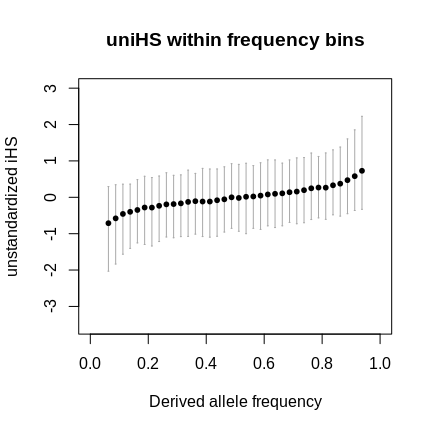Figure 5.1: Graphical output of the freqbinplot() function Note that this frequency dependence is expected under neutral evolution and not due to selection. In fact, an implicit assumption of the bin-wise standardization is that each bin is dominated by neutral markers. Thus, the bin-wise standardization reduces the dependency of iHS on the allele frequency; see section 7.6 of this vignette and Figure 4 in (Voight et al. 2006) [In their case the effect is even more pronounced, because they polarized alleles with help of an outgroup species while in the case of our data it was done using extant ancestral populations as a proxy.] ## 5.2Rsb vs. XP-EHH comparison Figure 5.2 shows a plot of Rsb against XP-EHH values across the CGU and EUT populations (see section 4.2.2 and 4.3.2 respectively). Marked in red is the marker that was repeatedly used in the examples above. The plot was generated using the following R code: plot(rsb.cgu_eut[, "RSB_CGU_EUT"], xpehh.cgu_eut[, "XPEHH_CGU_EUT"], xlab = "Rsb", ylab = "XP-EHH", pch = ".", xlim = c(-7.5, 7.5), ylim = c(-7.5, 7.5)) # add red circle for marker with name "F1205400" points(rsb.cgu_eut["F1205400", "RSB_CGU_EUT"], xpehh.cgu_eut["F1205400", "XPEHH_CGU_EUT"], col = "red") # add dashed diagonal abline(a = 0, b = 1, lty = 2)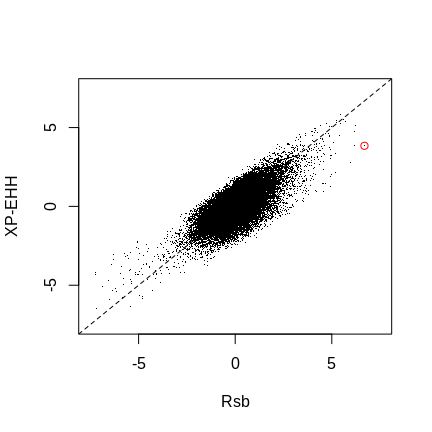Figure 5.2: Comparison of Rsb and XP-EHH values across the CGU and EUT populations ## 5.3 Distribution of standardized values: the function distribplot() The distribplot() function allows to visualize the distributions of the standardized scores (either iHS, Rsb or XP-EHH) and compare them to the standard Gaussian distribution. As an example, in Figure 5.3 the function was applied on the iHS estimates obtained for the CGU population (see section 4.1.1): distribplot(wgscan.ihs.cgu$ihs$IHS, xlab = "iHS")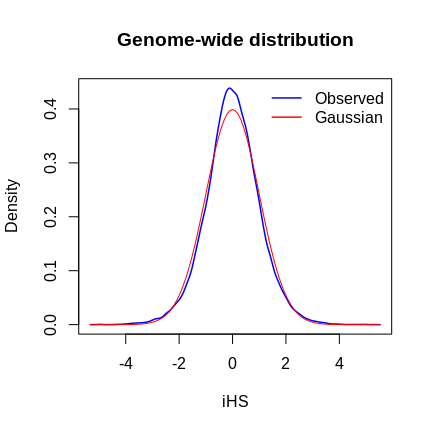Figure 5.3: Graphical output of the distribplot() function Setting the option qqplot to TRUE toggles the output to a qqplot, as in Figure 5.4. distribplot(wgscan.ihs.cgu$ihs$IHS, xlab = "iHS", qqplot = TRUE)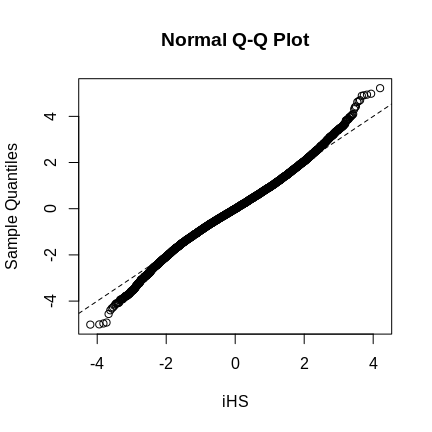Figure 5.4: Graphical output of the distribplot() function ## 5.4 Genome wide score plots: the function manhattanplot() The function manhattanplot() draws a Manhattan plot of the whole genome scores obtained by the functions ihh2ihs(), ines2rsb() or ies2xpehh(). Figure 5.5 was drawn using the command: manhattanplot(wgscan.ihs.cgu, main = "iHS (CGU cattle breed)")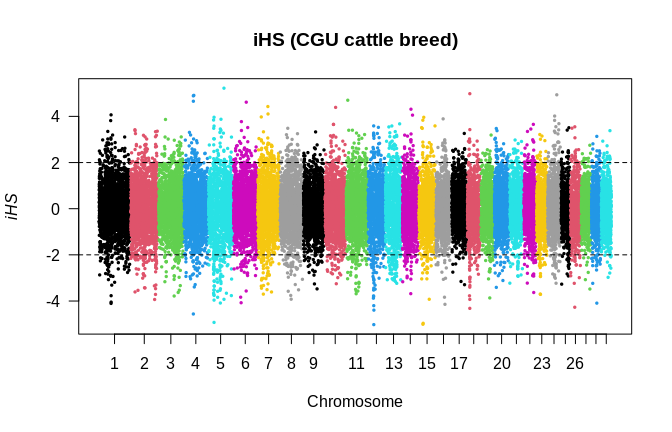Figure 5.5: Graphical output of the manhattanplot() function If the option pval is switched to TRUE the associated p-value instead of the score itself is plotted (Figure 5.6). manhattanplot(wgscan.ihs.cgu, pval = TRUE, threshold = 4, main = "p-value of iHS (CGU cattle breed)")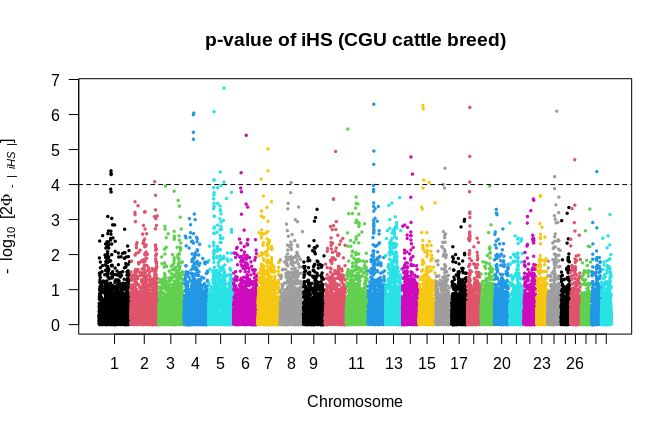Figure 5.6: Graphical output of the manhattanplot() function The colors of the chromosomes in Figures 5.5 and 5.6 are the default colors of R as obtained by the command palette(). It is possible to change them by the same command. Note that the colors are associated with the order of chromosomes in the scan and not their order in the plot. Candidate regions as obtained by the function calc_candidate_regions() can be added to the plot as parameter cr. Individual markers can be highlighted by setting argument mrk to a vector of marker IDs or a data frame with positions (containing columns with name CHR and POSITION). By default, chromosomes are separated by an inset of 5,000,000 bases. This value can be increased by the corresponding parameter in order to further reduce overlap between data points of neighboring chromosomes. In order to reduce the number of plotted data points, the data set can be rasterized in both dimensions by parameter resolution. The data points are then rounded to the specified resolution and duplicate points removed. Furthermore, it is possible to specify a subset or a re-ordering of chromosomes with help of parameter chr.name as in Figure 5.7. # re-define colors palette(c("red", "green")) manhattanplot(wgscan.ihs.cgu, pval = TRUE, threshold = 4, chr.name = c("1", "4", "5", "12"), main = "iHS (CGU cattle breed)", cr = cr.cgu, mrk = "F1205400", inset = 1E+7, resolution = c(200000, 0.05)) > Rasterization reduced 6958 data points to 6377 . # set back to default colors palette("default")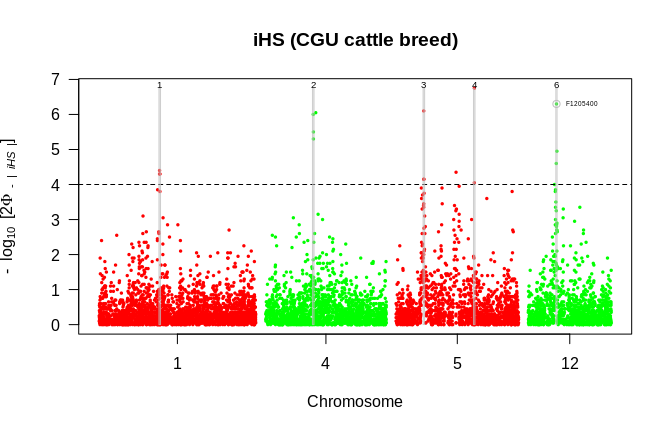Figure 5.7: Graphical output of the manhattanplot() function ## 5.5 Genome wide score plots: the function manhattan() of package qqman The package qqman contains a function manhattan() which is similar to the function manhattanplot() of this package. The input data frame is expected to have a slightly different format, though. Hence, before plotting we need to “translate” our data as in the following example with iHS values: # extract data frame from result list ihs <- wgscan.ihs.cgu$ihs
# create new data frame
wgscan.cgu.ihs.qqman <- data.frame(
CHR = as.integer(factor(ihs$CHR, levels = unique(ihs$CHR))),
# chromosomes as integers
BP = ihs$POSITION, # base pairs P = 10**(-ihs$LOGPVALUE),  # transform back to p-values
SNP = row.names(ihs)       # SNP names
)

We can now use the new data frame as input for the function manhattan() to obtain Figure 5.8:

library(qqman)
qqman::manhattan(wgscan.cgu.ihs.qqman,
col = c("red","green"),
chrlabs = unique(ihs\$CHR),
suggestiveline = 4,
highlight = "F1205400",
annotatePval = 0.0001)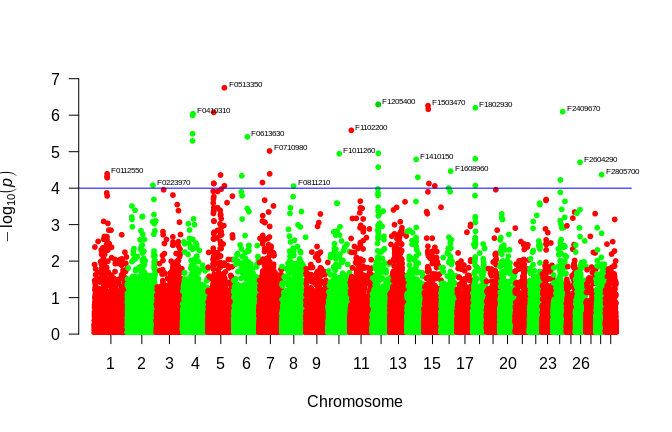Figure 5.8: Graphical output of the qqman::manhattan() function

# 6 Visualization of the haplotype structure

## 6.1 The function plot.haplohh()

Chunks of the haplotype matrix can be plotted, provided they are not too large, with sequences in rows and markers in columns. The following plot shows a chunk of 200 SNPs in the vicinity of SNP F1205400 which is identified by the dashed line. The derived alleles are marked in red, the ancestral ones not shown.

hh_subset <- subset(haplohh_cgu_bta12, select.mrk = 350:550)
oldpar <- par(mar = c(3, 2, 2, 2) + 0.1)
plot(
hh_subset,
mrk = "F1205400",
group_by_allele = TRUE,
ignore.distance = TRUE,
col = c(NA, "red"),
linecol = c("lightblue", "lightpink"),
mrk.col = "black",
cex = 0.1,
pos.lab.hap = "none",
pos.lab.mrk = "none"
)
par(oldpar)